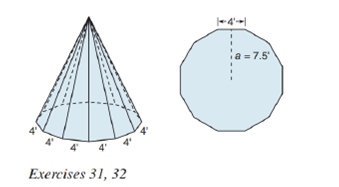Chapter 9.2, Problem 31E### Elementary Geometry for College St...

6th Edition
Daniel C. Alexander + 1 other
ISBN: 9781285195698

#### Solutions

Chapter
Section### Elementary Geometry for College St...

6th Edition
Daniel C. Alexander + 1 other
ISBN: 9781285195698
Textbook Problem
53 views

# A teepee is constructed by using 12 poles. The construction leads to a regular pyramid with a dodecagon (12 sides) for the base. With the base as shown, and knowing that the height of the teepee is 15 f t , find its volume.To determine

To find:

The volume of the pyramid.

Explanation

Given:

A teepee in shape of a regular pyramid with a dodecagon (12 sides) for the base as below,

and the height of the teepee is 15ft and side of the base as 4ft and apothem a=7.5ft.

Properties Used:

A pyramid is made by connecting a base to an apex. The base is flat with straight edges, no curves, hence, a polygon and all other faces are triangles.

A regular pyramid is a pyramid whose base is a regular polygon and whose lateral edges are all congruent.

The lateral area L of a regular pyramid with slant height of length l and perimeter P of the base is given by

L=12lP.

The total surface area T of a pyramid with lateral area L and base area B is given by

T=L+B.

According to the Pythagorean theorem, in a right-angled triangle

hypotenuse2=base2+perpendicular2.

In a regular pyramid, the lengths of the apothem a of the base, the altitude h, and the slant height l satisfy the Pythagorean Theorem; that is, l2=a2+h2.

The perimeter of the square is four times the side

### Still sussing out bartleby?

Check out a sample textbook solution.

See a sample solution

#### The Solution to Your Study Problems

Bartleby provides explanations to thousands of textbook problems written by our experts, many with advanced degrees!

Get Started

#### Find more solutions based on key concepts